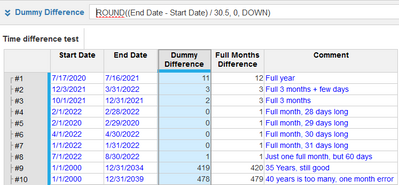# Calculate the number of complete months between two dates (Similar to Excel DATEDIF Function)Hello Community!

Is there a way to calculate the number of complete months between two dates in Anaplan?

E.g.

 Start Date (MM/DD/YYYY) End Date (MM/DD/YYYY) Number of Complete Months 07/17/2020 07/16/2021 12 12/03/2021 03/31/2022 3 10/1/2021 12/31/2021 3

In the second row, even though the duration between 12/03/2021 & 03/31/2022 is 3 months, 29 days - the system should return 3 as the number of complete months.

Any help on the best way to solve this would be appreciated.

Thanks.

Tagged:

•Hi @sosunkwo,

The answer greatly depends on how you define this difference. Using your 3 examples, I tried to produce something that might be close (with more examples covering edge cases):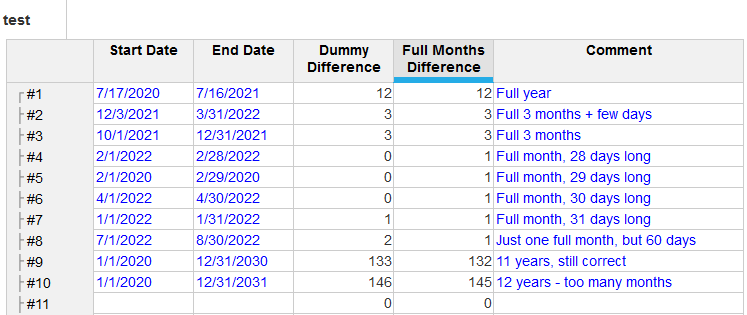Blueprint: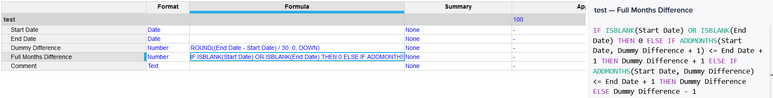•@sosunkwo You may try something below

(End Date - Start Date) / 30

or there is similar ask here ,see if this works

https://community.anaplan.com/t5/Anaplan-Platform/How-to-Calculate-Months-Difference-between-Two-Dates/m-p/849#M3585

•Give this is shot...

Create a module (Start/End Month) that is dimensionalized by what you are trying to figure out, I used Row Count but this could very well be Invoice ID or SKU.  Line items Sart and End are both formatted as Date.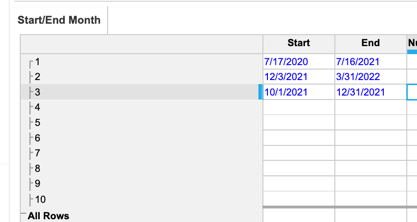In another module, use the same list as above and add the Time list (at the month level) with the lone line item being Complete Month, formatted as number with Summary turned to None.  The formula should be:

IF START() >= 'Start/End Month'.Start AND END() <= 'Start/End Month'.End THEN 1 ELSE 0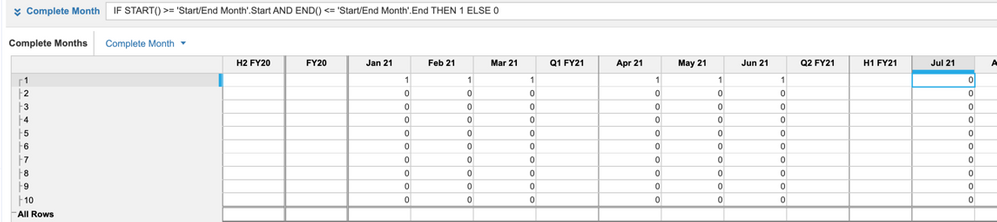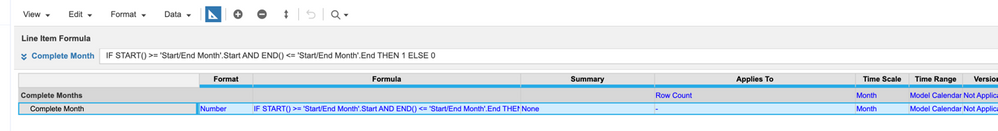Back in your Start/End Month module (the first one), create another line item named Number of Complete Months, formatted as Number with Summary set to None.  For the formula, you can use TIMESUM(Complete Months.Complete Month) since this module does not have Time in the Applies To.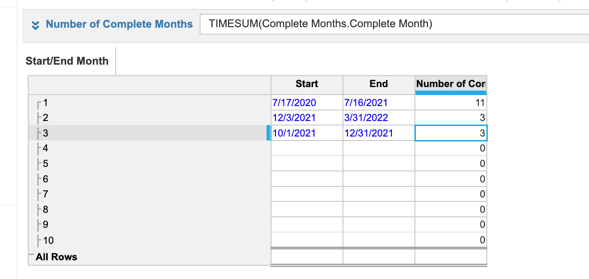If you have All Periods turned on in your module, you and use Complete Months.Complete Month[Select: Time.All Periods]

Hope this helps,

Rob

•Did the above "solve" your issue?

Rob

•Thanks, @bhatjaved. I tried this approach and found it doesn't always give accurate results. E.g. 12/3/2021 - 3/31/2021 outputs as 4 months instead of 3 months.
And if I added the ROUND DOWN function to it, some results will be inaccurate. E.g. 2/1/2022 - 3/31/2022 will become 1 month instead of 2 months.

•Hi, @rob_marshall, thanks a lot for your detailed response.

Though it functions as you described, it is not exactly what I'm looking for because the formula is not inclusive of situations where the start & end dates are not the first and last days of the months, respectively.
E.g. in your screenshot example, 7/17/2020 - 7/16/2021 returns 11 months instead of 12 months.

Another example, let's say Start Date: 1/15/2022 and End Date: 3/14/2022:

START(): Jan 1, 2022 >= Jan 15, 2022 = FALSE AND END(): Jan 31, 2022 <= Mar 14, 2022 = TRUE

START (): Feb 1 , 2022 >= Jan 15, 2022 = TRUE AND END(): Feb 28, 2022 <= Mar 14, 2022 = TRUE

START (): Mar 1, 2022 > = Jan 15, 2022 = TRUE AND END(): March 31, 2022 <= Mar 14, 2022 = FALSE

The only month scenario where both conditions are being met is scenario 2 (green text), so the count of complete months comes out as one month. But it should be two months because technically, Jan 15, 2022 - March 14, 2022, is two months.

*The START() function returns the first date of a time period.

* The END() function returns the last date of a time period.

I also found that the count excludes months that fall outside of the model calendar - which makes sense seeing that the intermediate module is dimensioned by the model calendar.

•Hi @M.Kierepka , thank you for the response. The solution you described works for me (including edge cases).

Would you mind explaining the logic? (i.e. the Dummy Difference & the Full Months Difference formulas).

•Hi,

1. Dummy Difference is something that gives you a rough estimate of how many months has passed - it's just working on the assumption that on average a month has 30 days, and we round it down to whole numbers, so it represents only full months. You can say it's heuristic, and even better one would be if you change 30 to 30.5.
2. Then, in Full Months Difference I use that rough estimation as base, but check if it's really correct, and if not, try to fix it by adding or subtracting one month, and again checking if maybe now it's right. That's because in Anaplan we can only estimate the difference in months, but we can precisely check if this value is correct.